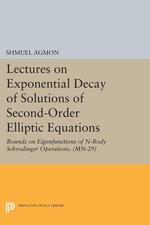# Lectures on Exponential Decay of Solutions of Second-Order Elliptic Equations: Bounds on Eigenfunctions of N-Body Schrodinger Operations. (MN-29)

Shmuel Agmon
Pages: 124
https://www.jstor.org/stable/j.ctt13x1d8z

1. Front Matter
(pp. [i]-[ii])
(pp. 1-2)
3. Chapter 0 Introduction
(pp. 3-10)

This volume presents an edited and slightly revised version of notes of lectures given at the University of Virginia in the fall of 1980. The subject of these lectures is the phenomenon of exponential decay of solutions of second order elliptic equations in unbounded domains. By way of introduction we discuss briefly the special problem of exponential decay of eigenfunctions of Schrödinger operators, a problem which motivated the present investigations.

Consider the Schrödinger differential operatorP= − ∆ +V(x) on${{\mathbb{R}}^{n}}$whereVis a real function in$L_{loc}^{1}({{\mathbb{R}}^{n}})$. Assume also that${{V}_{-}}\in L_{loc}^{p}({{\mathbb{R}}^{n}})$for some p > n/2...

4. Chapter 1 The Main Theorem
(pp. 11-31)

Let Ω be a connected open set in${{\mathbb{R}}^{n}}$. We consider in Ω a second order elliptic operatorA(x ,∂) of the form

$A(x,\partial )=-\sum\limits_{i,j=1}^{n}{{{\partial }_{i}}{{a }^{ij}}(x){{\partial }_{i}}}$ (1.1)

whereaij(x) are continuous real valued functions and the matrix [aij(x)] is positive definite for everyx∊ Ω. We shall consider the equation

$A(x,\partial )u(x)+q(x)u(x)=f(x)$ (1.2)

for complex valued functions$q\in L_{loc}^{1}(\Omega )$and$f\in L_{loc}^{2}(\Omega )$. Since the coefficientsaij(x) are not required to be differentiable the equation (1.2) should be interpreted in a generalized sense. In this connection it is convenient to introduce the following notation:

1.1 Definition: Let$u,v\in H_{loc}^{1}(\Omega )$. Then we define

${{\nabla }_{A}}u(x)\cdot {{\nabla }_{A}}v(x)=\sum\limits_{i,j=1}^{n}{{{a}^{ij}}(x){{\partial }_{i}}u(x){{\partial }_{j}}v(x),}$ (1.3)

${{\left| {{\nabla }_{A}}u(x) \right|}^{2}}={{\nabla }_{A}}u(x)\cdot {{\nabla }_{A}}\overline{u(x)}=\sum\limits_{i,j=1}^{n}{{{a}^{ij}}(x){{\partial }_{i}}u(x){{\partial }_{j}}}\overline{u(x)}.$ (1.4)...

5. Chapter 2 Geometric Spectral Analysis
(pp. 32-40)

Our goal is to apply Theorem 1.5 to measure the decay of solutions toAu+qu=0. Ifq(x) =q1(x) −μwe thereby obtain estimates for eigenfunctions ofA+q1with eigenvalueμ. To apply Theorem 1.5 the real part of the quadratic form associated with the operatorA+qneed be positive on$C_{0}^{\infty }(\Omega )$. Moreover, we must find a positive continuous function λ(x) such that the quadratic form in question is strongly positive on$C_{0}^{\infty }(\Omega )$in the sense that the λ-Condition (1.7) holds. (Since we are dealing with behavior of solutions “at infinity” the inequality (1.7)...

(pp. 41-51)

In this chapter we consider the realization ofP=A+qas an operator on${{L}^{2}}({{\mathbb{R}}^{n}})$. We show that when Σ(P) >− ∞Ptogether with a suitable domain define a self-adjoint operatorHwhich is bounded from below. We also find that Λ(P) and Σ(P) are equal to the infimum of the spectrum and essential spectrum ofHrespectively. The results of this chapter will allow us to apply exponential decay results to eigenfunctions ofH.

Throughout this chapter we assume thatPsatisfies the conditions imposed onP=A+qin Chapter 2. That is,$A=-\sum\limits_{i,j}{{{\partial }_{j}}{{a}^{ij}}{{\partial }_{i}}}$where...

7. Chapter 4 L2 Exponential Decay Applications to eigenfunctions of N-body Schrodinger operators
(pp. 52-82)

In this chapter we apply Theorem 1 5 to proveL2exponential decay results In Theorem 4 1 we consider the general class of elliptic operatorsA+qstudied in Chapter 2 and Chapter 3 without taking into account in a detailed way the variation of Λ(x,R) in distant regions of${{\mathbb{R}}^{n}}$Thus replacing Λ by a constant we prove the theorem using a metricρλwith λ = constant In Theorem 4 4 we consider operatorsA+qwith constantaij, but take into consideration the variation of Λ(x,R) with direction for large$\left| x \right|$by making use of the...

8. Chapter 5 Pointwise Exponential Bounds
(pp. 83-98)

In this chapter we will derive pointwise bounds for solutions of the equationAu+qu= 0 in a bounded subset of${{\mathbb{R}}^{n}}$. When combined with the results of Chapter 4 these bounds will give pointwise exponential decay bounds for eigenfunctions of theN-body Schrödinger Hamiltonians considerd in Theorem 4.13, as well as pointwise exponential decay bounds for eigenfunctions of the atomic type Schrödinger Hamiltonians considered in Theorem 4.12 and for eigenfunctions of the general multiparticle type Schrödinger operators considered in Theorem 4.9.

Pointwise bounds for solutions of the equationAu= 0 were derived in the well known...

9. Appendix 1 Approximation of Metrics and Completeness
(pp. 99-101)
10. Appendix 2 Proof of Lemma 1.2
(pp. 102-103)
11. Appendix 3 Proof of Lemma 2 2
(pp. 104-109)
12. Appendix 4 Proof of Lemma 5.7
(pp. 110-111)